## The Pacific Ring of Fire is a major area in the basin of the Pacific Ocean where many earthquakes and volcanic eruptions occur. In a large horseshoe shape, it is associated with a nearly continuous series of oceanic trenches, volcanic arcs, and volcanic belts and plate movements.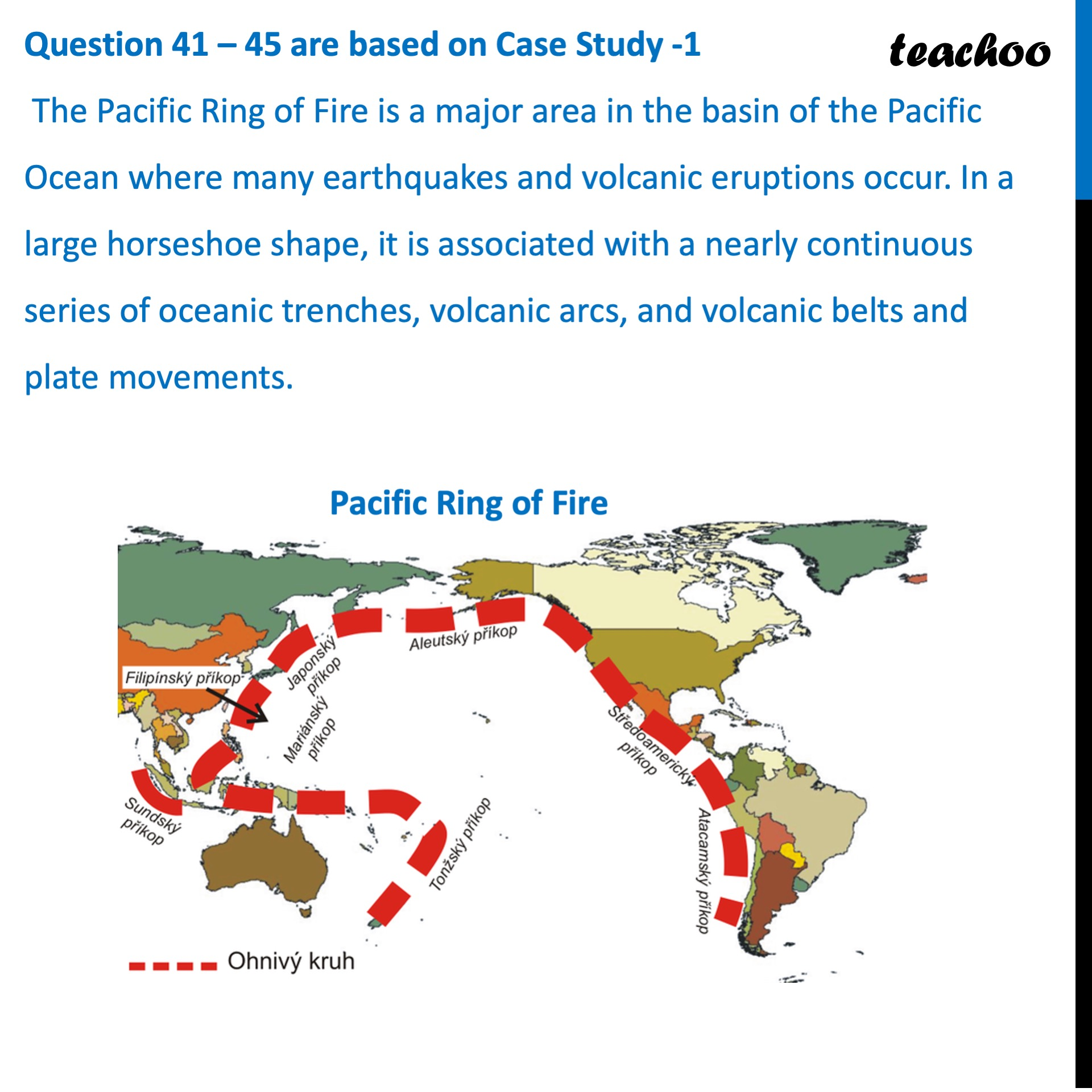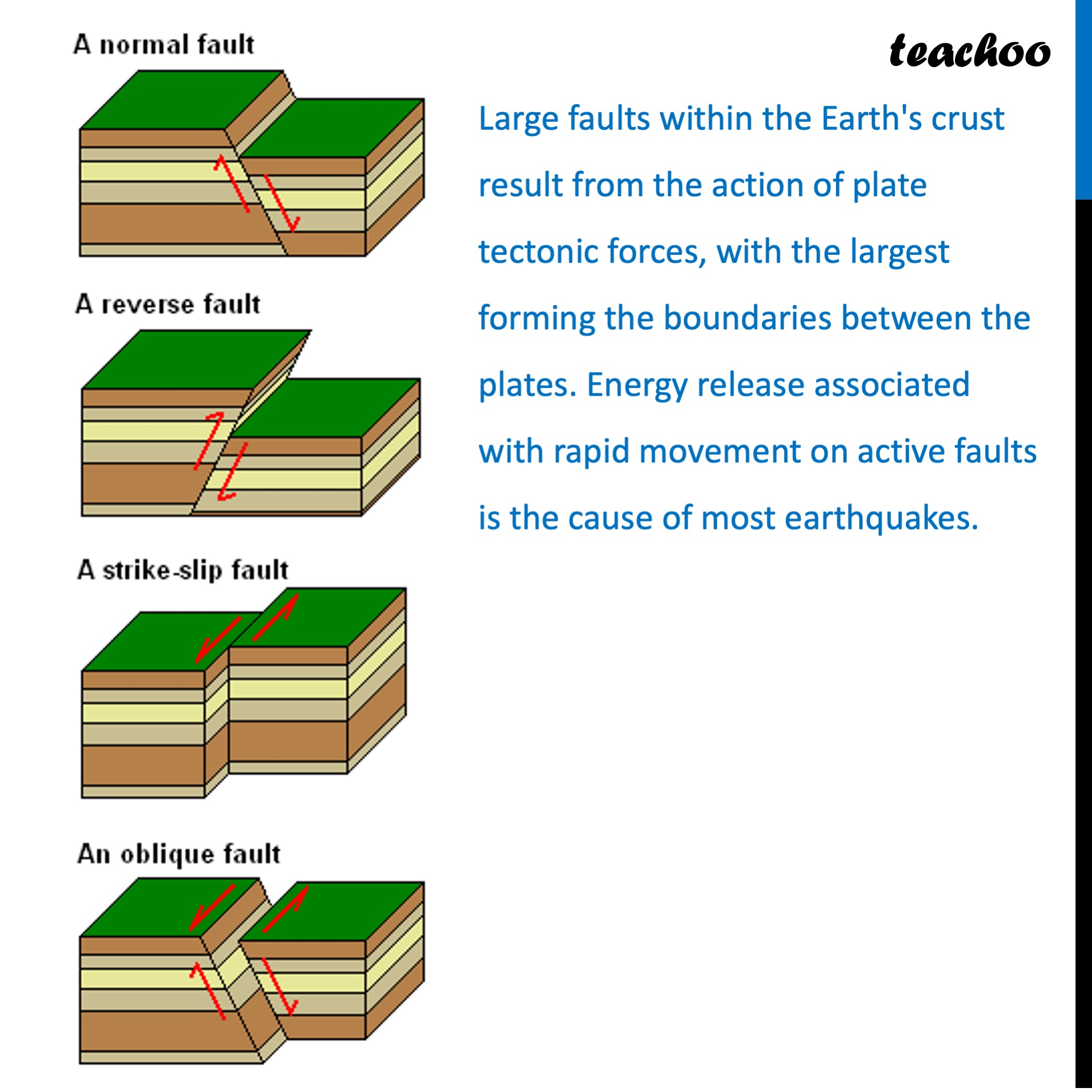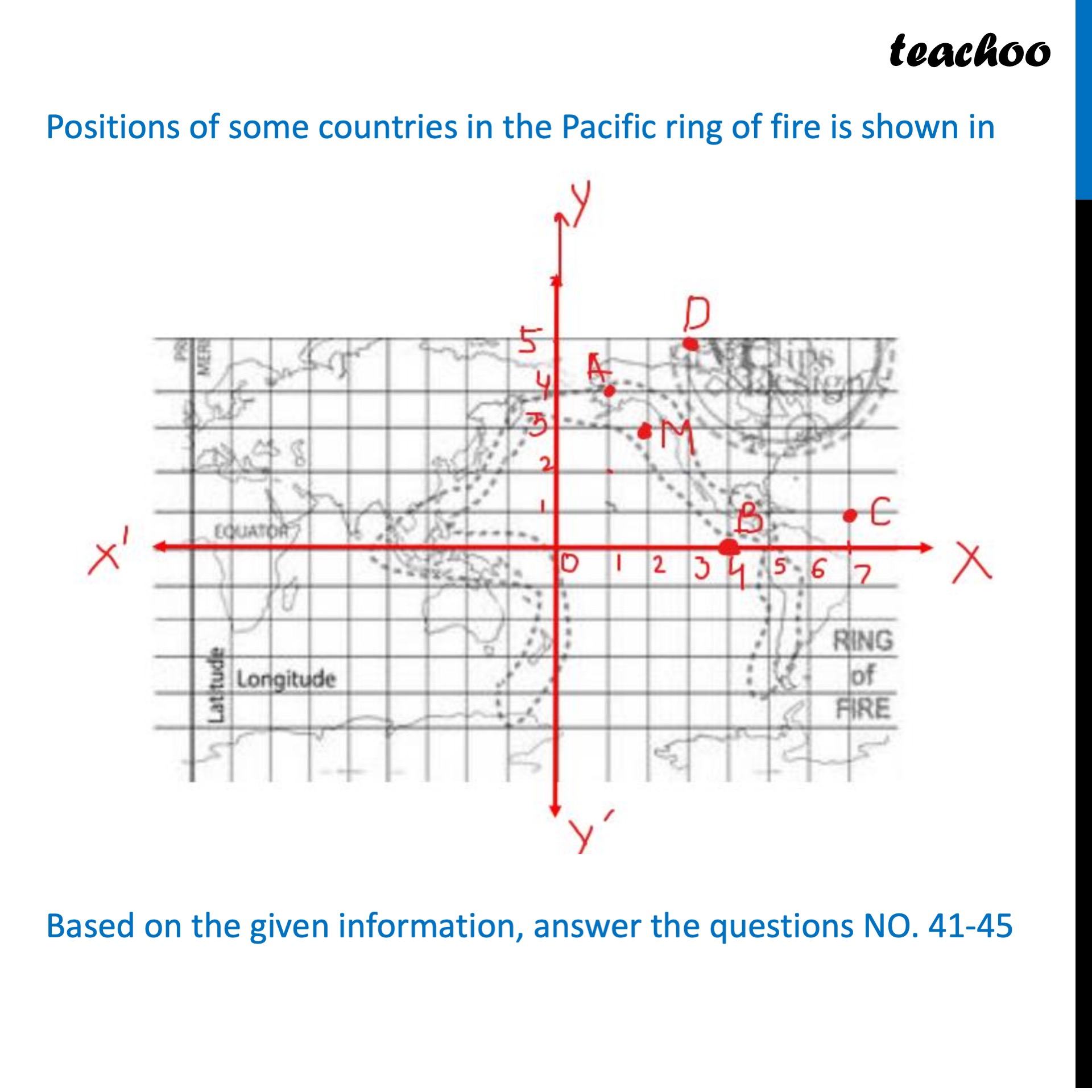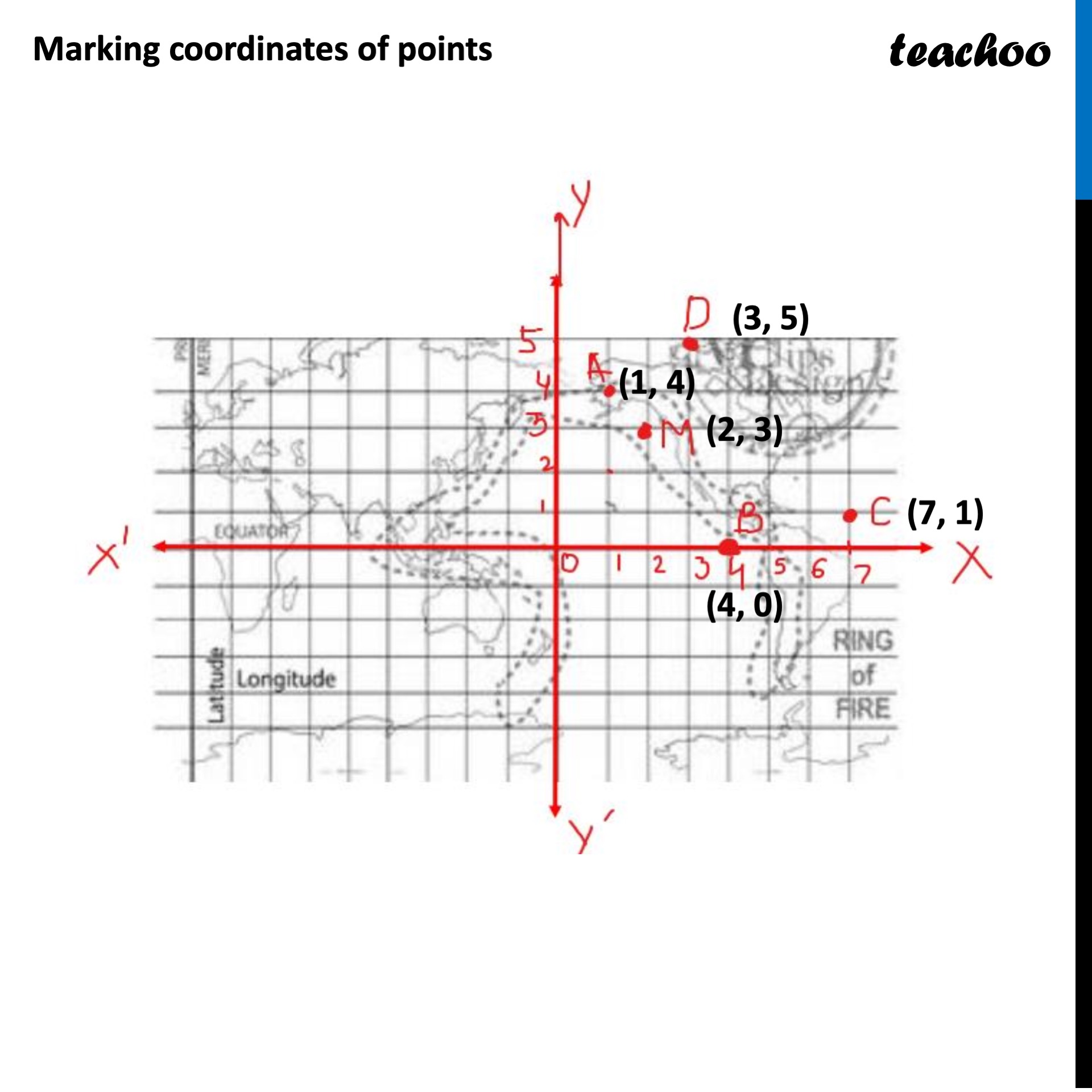### The distance between the point Country A and Country B is (a) 4 units   (b) 5 units   (c) 6 units   (d) 7 units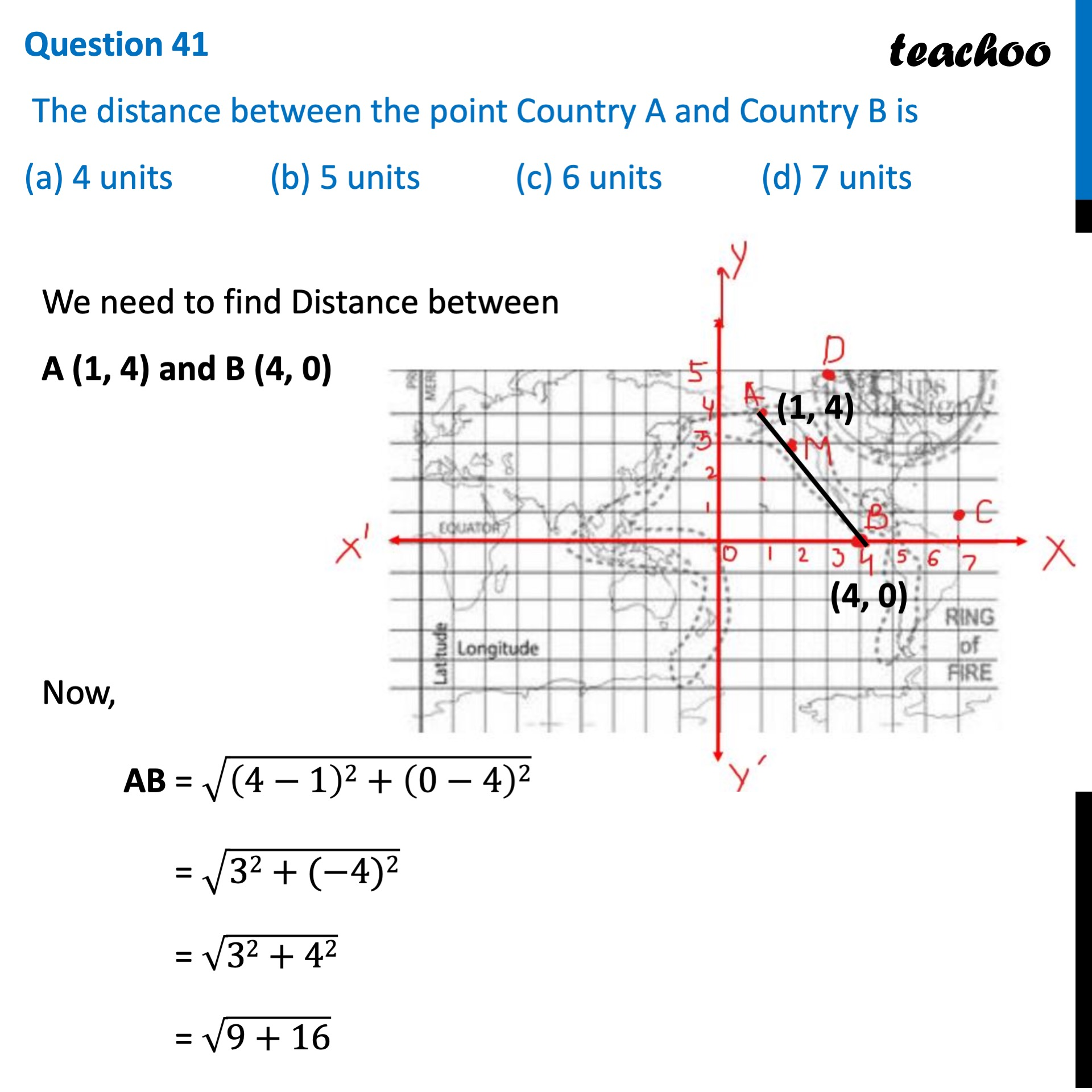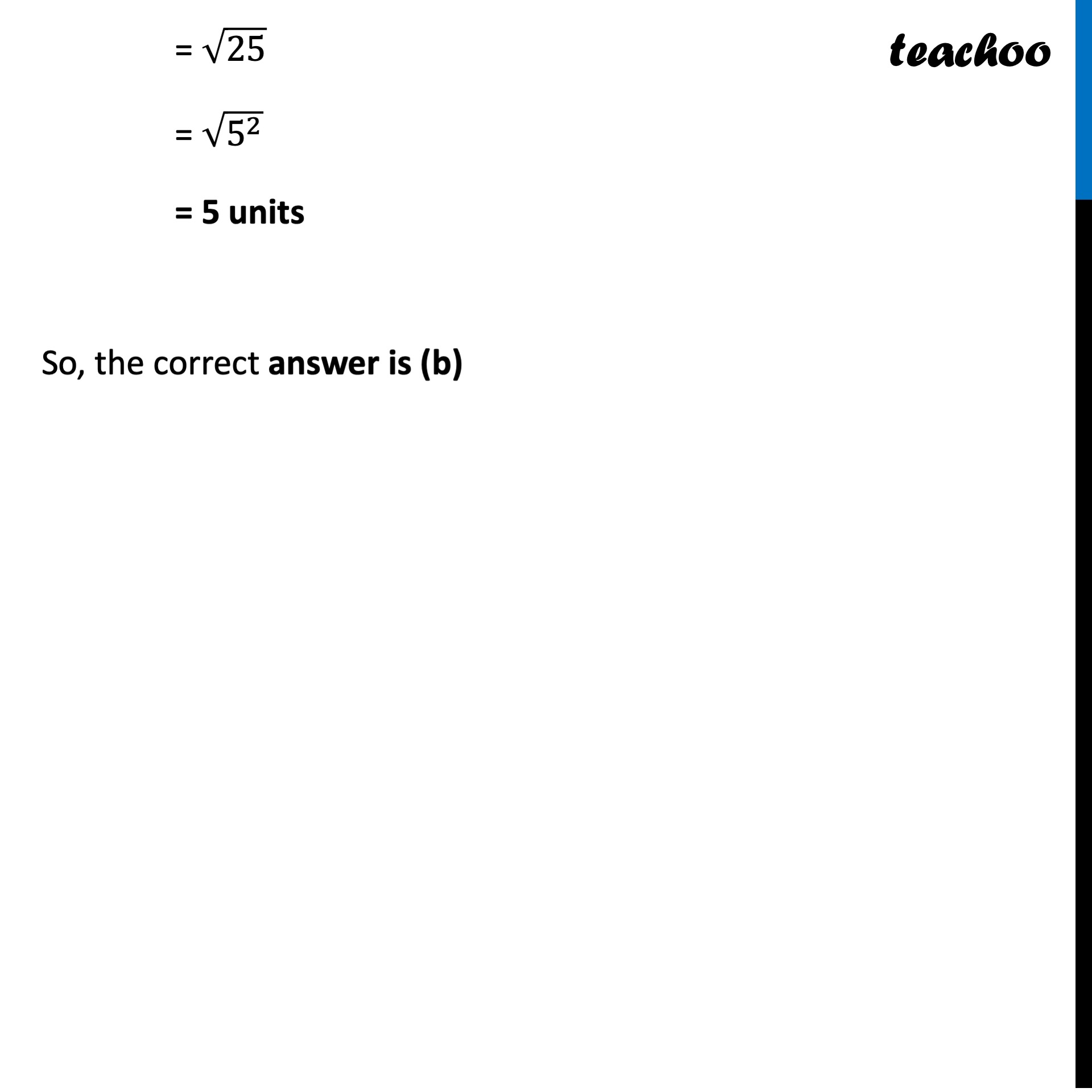1. Class 10
2. Solutions of Sample Papers for Class 10 Boards
3. CBSE Class 10 Sample Paper for 2022 Boards - Maths Basic [MCQ]

Transcript

Question 41 – 45 are based on Case Study -1 The Pacific Ring of Fire is a major area in the basin of the Pacific Ocean where many earthquakes and volcanic eruptions occur. In a large horseshoe shape, it is associated with a nearly continuous series of oceanic trenches, volcanic arcs, and volcanic belts and plate movements. Large faults within the Earth's crust result from the action of plate tectonic forces, with the largest forming the boundaries between the plates. Energy release associated with rapid movement on active faults is the cause of most earthquakes. Positions of some countries in the Pacific ring of fire is shown in Based on the given information, answer the questions NO. 41-45 Question 41 The distance between the point Country A and Country B is (a) 4 units (b) 5 units (c) 6 units (d) 7 units We need to find Distance between A (1, 4) and B (4, 0) Now, AB = √((4−1)2+(0−4)2) = √(32+(−4)2) = √(32+42) = √(9+16) = √25 = √(5^2 ) = 5 units So, the correct answer is (b)